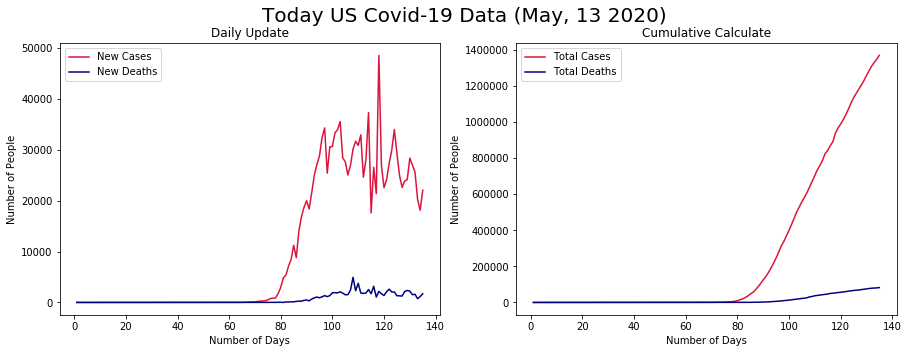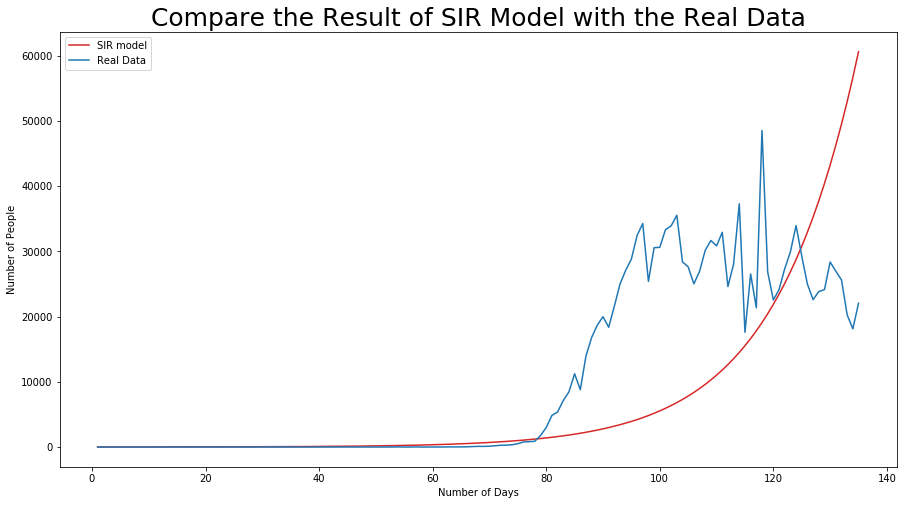# 2019-nCoV epidemic and thinking of the existing SIR model¶

## Abstract¶

In this project, we first use sample testing to evaluate the rationality and practicality of the 2019-nCoV model, and then analyze the commonality of infectious diseases and the characteristics of 2019-nCoV. A single basic assumption is made, and the crowd is ideally divided into three categories (S, I, R) according to the content of the lecture, and a basic SIR model is established. Due to the characteristics of the virus, it can be seen that the two important parameters isolation and reinfect index of the SIR model are values with time as a variable. Based on the real-time statistical data of the daily epidemic situation in the United States and the model, we can obtain the approximate values of isolation and reinfect index. Due to the gradual improvement of medical conditions, a set of effective treatment plans will surely be developed, and even later vaccine development. Therefore, without changing the population classification, a coefficient c is added to represent the success rate of the treatment plan. Since the death of the population and the complications caused by the epidemic are considered, c is set as a constant. We hope that the new model analyzed in combination with the display data will reflect the changing law of various types of people. If the previous period is consistent with the change, it will be regarded as meeting the basic needs of our model.

## Model Constract¶

Mainly by analyzing the changes in the number of infected people in the United States, we propose the following model assumptions for the prediction of the trend of epidemic changes in the region:

1. Divide the crowd into three categories:
• Susceptible number (suspected cases): expressed as S;
• Number of patients (infected persons, ie diagnosed): expressed by I;
• Number of people moved out (including "healed" and "dead"): Represented by R.
2. The population in this area is not mobile (considering that the United States has been in a shutdown state for most of the week, this assumption is reasonable), suppose the initial number of vulnerable people is N, at this time I, R are 0.
3. The quarantined people completely cut off contact with the outside world and are no longer infectious (this assumption is also reasonable considering the existing medical conditions).
In :
# import libraries
import pandas as pd
from numpy import *
from pylab import *
from datetime import *

In :
df = pd.read_csv("https://covid.ourworldindata.org/data/owid-covid-data.csv")
df = df.loc[df['location'] == "United States"]
df = df.drop(columns=['iso_code','location'])
d = datetime.now().strftime('%b, %d %Y')
t = linspace(1, len(df['new_cases']), len(df['new_cases']))
figure(figsize=(15,5))
subplot(121)
plot(t, df['new_cases'], 'crimson', label='New Cases')
plot(t, df['new_deaths'], 'navy', label='New Deaths')

# plot(t, df['new_tests'],'yellowgreen', label='New Test')
xlabel('Number of Days')
ylabel('Number of People')
title('Daily Update')
legend()
subplot(122)
plot(t, df['total_cases'], 'crimson', label='Total Cases')
plot(t, df['total_deaths'], 'navy', label='Total Deaths')
# plot(t, df['total_tests'],'yellowgreen', label='Total Tested')
xlabel('Number of Days')
ylabel('Number of People')
title('Cumulative Calculate')
legend()
suptitle('Today US Covid-19 Data (' + d + ')', fontsize=20)
show()It is preferred to use pandas to summarize daily data (data sources see the reference statement below) into a dataframe. After that, we only take the data from the United States to analyze and find that the data in the first half (about sixty days from the beginning) has basically no change, so the data in this period is ignored.

In :
rate = df['new_cases'].iloc[-1:] / df['population'].iloc[-1]
float(rate * 100)
df['total_tests'].max()

Out:
9348458.0
In :
def sir(g):
T = len(df['date'])
N = int(df['total_tests'].max())
S = zeros(T)
I = zeros(T)
R = zeros(T)
I = df['new_cases'].iloc
S = N - I
R = N - S - I
dt = 1
time = zeros(T)
gamma = 1/g
Ro = 2
beta = Ro * gamma
m = I
day = 0
for d in range(1, T):
time[d] = d
S[d] = S[d-1] - dt * beta * I[d-1] * S[d-1] / N
I[d] = I[d-1] + dt * (beta * I[d-1] * S[d-1] / N - gamma * I[d-1])
R[d] = N - S[d] - I[d]
if I[d] >= m:
m = I[d]
day = d
return I
figure(figsize=(15,8))
plot(t, sir(14),'tab:red', label='SIR model')
plot(t, df['new_cases'],'tab:blue', label='Real Data')
legend()
xlabel('Number of Days')
ylabel('Number of People')
title('Compare the Result of SIR Model with the Real Data', fontsize= 25)
show()In the model, we use the incident angle distance as the recommended two weeks, and set the total population as the total number of detections, otherwise results may be too high. From the figure, we can see that because this SIR only considers the infection rate, the other values are derived from the number of infected people, so the predicted result has a relatively large error with the actual result.

# Problem of the Model and Consideration¶

## Problem analysis¶

Although the improved model can make an ideal trend analysis of the epidemic situation, the treatment of the late epidemic situation still deviates from the real situation. It is mainly caused by three reasons. First, the SIR model is too streamlined, and the real situation is over-idealized. The infection in this outbreak is not only transmitted by the infected person. For some susceptible people, as long as they carry pathogens, they can be used as the source of infection, but this part of the population has not been reflected in the establishment of the model. Secondly, the epidemic situation is still in the rising period, and the real data is insufficient. We have no way to make a good fit before the control. Similarly, we do not know about it after the control. The third is that the United States has a large number of bases, and due to improper control at the early stage of the outbreak, population movement has been greater. This is a great challenge for the establishment of the model, because the general model requires a fixed population and no population communication.

The following mainly explains the problems in this case from the perspective of model establishment. Refer to the more reasonable mathematical model established by the SARS epidemic.

## More reasonable model building process¶

1. Assuming that the total number of people under investigation is constant, and there is no source of input and output
2. Divide the surveyed population into normal people (susceptible people), diagnosed patients (infectors), withdrawn (cured and died), suspected patients (quarantined but not yet diagnosed)
3. Assume that the cured patient will not be infected again
4. Assuming that all patients are "others imported patients", that is, the individual's own disease is not considered
5. Assume that all types of people are evenly distributed among the people
6. Assume that there will be no cross-infection between people who have been quarantined
7. Regardless of the hidden virus carriers, that is, as long as they carry the virus, they can get sick.
• $I$: Confirmed patient
• $E$: Latent patient (infected person who is in latent and infectious)
• $R$: Withdrawal
• $S$: Ordinary susceptible
• $a_1$: Patient's infection coefficient
• $a_2$: Infection coefficient of patients in incubation period
• $d_1 - d_2$: The incubation period of the virus
• $d_3$: Cure time of sick patients
• $r$: The number of people per day in this group
• $p$: The controllable parameter is the intensity of isolation measures (the percentage of patients isolated during the incubation period)
• $w$: Proportion of people diagnosed who still have the opportunity to be infected
\begin{align} \frac{dS}{dt} & = -a_1wIr - a_2(1-p)Er \\ \frac{dE}{dt} & = a_1wIr + a_2(1-p)Er - \frac{2}{d_1+d_2}E \\ \frac{dI}{dt} & = \frac{2}{d_1+d_2}E-\frac{1}{d_3}I \\ \frac{dR}{dt} & = \frac{1}{d_3}I \end{align}